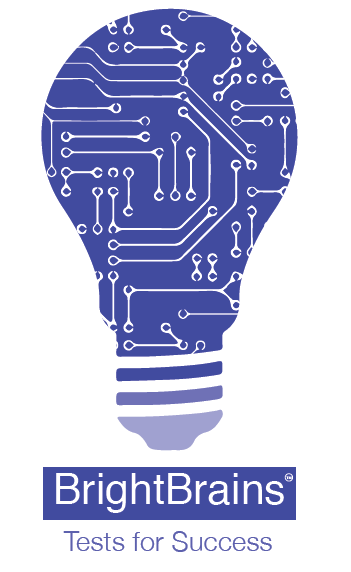www.brightbrains.in

 DataStructure Level:-Basic Q(1) Key value pair is usually seen in _______. AHash tables BHeaps CSkip list DBoth A and B Q(2) The linked list can be implemented using arrays. ATrue BFalse Level:-Intermediate Q(1) The array that represents a binary max-heap is _______. A{25, 12, 16, 13, 10, 8, 14} B{25, 14, 16, 13, 10, 8, 12} C{25, 14, 12, 13, 10, 8, 16} D{25, 14, 13, 16, 10, 8, 12} Q(2) In tree construction, which is the suitable efficient data structure? AArray BLinked list CStack DQueue Level:-Expert Q(1) Merge sort uses ______. ADivide and conquer strategy BHeuristic search CBacktracking approach DGreedy approach Q(2) Sparse matrices have ________. Ano zero Bmany zero Chigher dimenstion Dnone of these